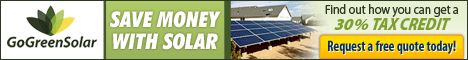# Solar Panel Calculator## Energy and Cost Calculations – Solar Panel Systems

Important!

Using these energy calculator provides rough figures. You should use the results to get a rough estimate of the size and cost of your required system. To get more accurate information it is advised that you consult an accredited renewable energy supplier.For information about how solar panels work, see the Solar Panels article this web site.

Also, be sure to stay on top of your country’s renewable energy subsidy programs as this will make a very big difference to the ultimate costs (see Subsidy Programs this web site).

Solar Panel System Calculators

The objective of using a solar panel system calculator is to determine the size, cost and eventual cost savings of the solar panel array you will need based on your electricity requirements and access to sunlight. To best understand how these calculations are made it is advised to have a look at the ‘Terms’  section below.

Solar Panel Energy Calculator

Terms

Capacity Factor

The capacity factor is a general term for all power generating systems and refers to the difference between what the system can achieve at continuous 100% output (the power rating) versus what it actually achieves under normal (less than 100%) operating conditions. The capacity factor for solar panels varies between 15% and 40%. This means that if a solar panel has a capacity factor of 25% it’s average energy output will be 25% of what it was designed to achieve, e.g., a 100 watt solar panel with a capacity factor of 25% has an average energy output of 25 watts. In the case of solar panels the capacity factor relates strongly to the insolation variable (see below).

Tolerance rating

A solar panel tolerance rating falls into two categories: Peak Tolerance Rating and Negative Tolerance Rating. Both of these variables relate to the amount of power (watts) that a new solar panel can be off specification, either higher or lower. The tolerance rating is solar panel specific and is not included as a variable in the calculators, therefore you will need to consult the manufacturer or a supplier to obtain this information

Insolation

Measured in kWh/m2 /day, insolation is the average amount of sunlight (solar radiation) per day at your location. This variable is necessary to get an approximate calculation of the total amount of energy a solar panel system will generate. This link can help you find the average amount of sunlight per day per year for your country.

DC Rating

Solar panels create DC electricity and are labeled in watts. This power rating is based on Standard Test Conditions (STC) for a single solar panel, and assumes optimum conditions. Therefore, to get the DC rating of an array, you need to add up the number of individual solar panels in the array and then multiply by the DC Rating of each panel, e.g.,

(20 solar panels)(DC Rating of 200 watts per solar panel) =  4000 watts

If you then divide this number by 1000 you can get kilowatts (kW), e.g., 4 kW. Kilowatts are necessary to compare with your conventional electricity bill.

Derate factor

The Derate factor is the loss of power efficiency due to all the factors that interfere with a perfect system. This includes factors such as the DC to AC Derate factor, age, shading, sun tracking, etc. The DC to AC Derate factor involves the conversion of DC electricity to AC electricity because solar panels create DC electricity and your house uses AC electricity (see Electricity article this website). Converting DC to AC requires additional devices including a transformer, DC and AC wiring, connections, etc.

Array type

Solar panel array type depends on the axis of rotation. There are three types:

• Fixed (default)
• sun-tracking with one axis of rotation
• sun-tracking with two axes of rotation

When solar panels track the sun they generate more electricity.

Tilt angle

The tilt angle is based on setting the horizontal angle ( _ I) of the solar panels’ plane so that it has maximum perpendicularity to the rays of the sun. This is dependent on latitude and time of year.

Azimuth angle

The azimuth angle is based on setting the vertical angle of the solar panels’ plane between east and west so that it has maximum perpendicularity to the rays of the sun. This is dependent on latitude, e.g., in the northern hemisphere the default azimuth angle is due south, and for the southern hemisphere it is due north. When calculating the azimuth angle it is important to use true north readings, as opposed to magnetic north ones.